Courses

# Previous Year Questions - Statistics Class 10 Notes | EduRev

## Class 10 : Previous Year Questions - Statistics Class 10 Notes | EduRev

The document Previous Year Questions - Statistics Class 10 Notes | EduRev is a part of the Class 10 Course Mathematics (Maths) Class 10.
All you need of Class 10 at this link: Class 10

Short Answer Type Questions

Q.1. The following data gives the information on the observed lifetimes (in hours) of 225 electrical components: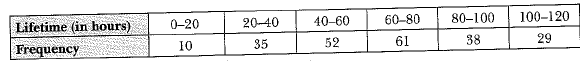Determine the modal lifetimes of the components.    [CBSE 2019 (30/5/1)]
Ans.
Here, the maximum class frequency is 61 and the class corresponding to this frequency is 60-80. So, the modal class is 60-80.
Here, l = 60, h = 20 ,f1 = 61, f0 = 52, f2 = 38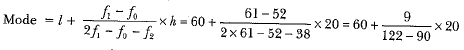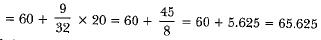Hence, modal lifetime of the components is 65.625 hours

Q.2. The table below shows the salaries of 280 persons.    [CBSE 2018]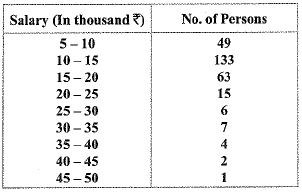Calculate the median salary of the data
Ans.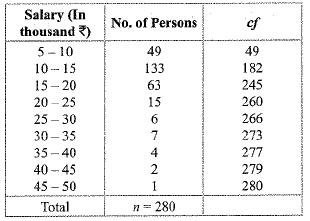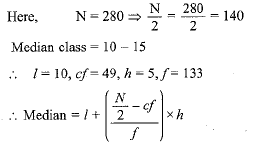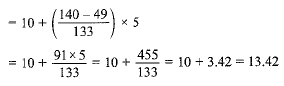Therefore, median salary = 13.42 (in thousand Rs.)

Q.3. By changing the following frequency distribution ‘to less than type’ distribution, draw its ogive.   [CBSE 2018 (C)]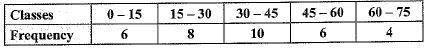Ans.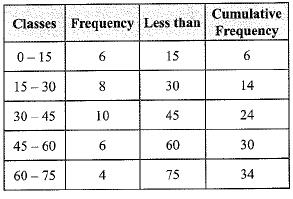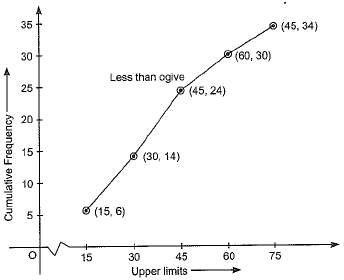Long Answer Type Questions

Q.1.  If the median of the following frequency distribution is 32.5. Find the values of f1 and f2.    [Delhi 2019]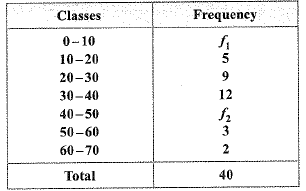Ans.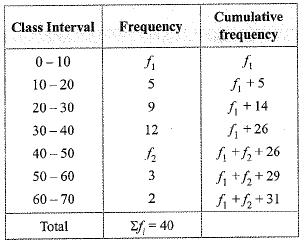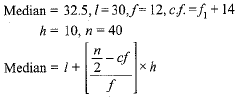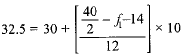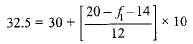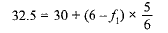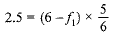⇒ 15 = 5(6 - f1 )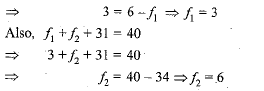Q.2. The following table gives the literacy rate (in percentage) of 35 cities. Find the mean literacy rate    [CBSE 2019 (30/5/2)]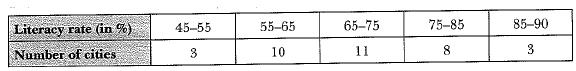Ans.
Here, we use step deviation method to find mean.
Let assumed mean A = 70 and class size h = 10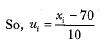Now, we have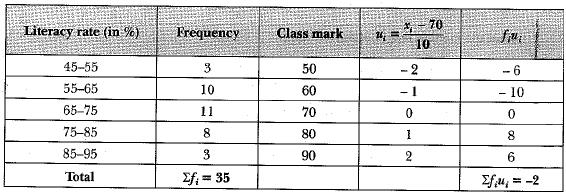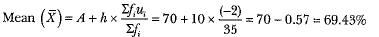Q.3. The following distribution shows the daily pocket allowance of children of a locality. The mean pocket allowance is Rs 18. Find the missing frequency f.    [CBSE 2019 (30/1/2)]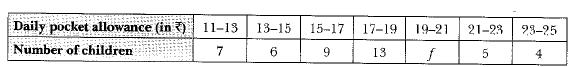Ans.
Let the assumed mean A = 16 and class size h = 2, here we apply step deviation method.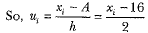Now, we have,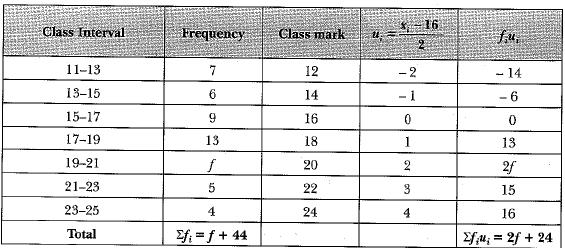We have, mean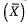= 18, A = 16 and h = 2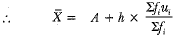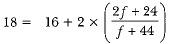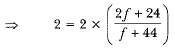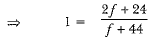⇒ f + 44 = 2f + 24
⇒ f = 44 - 24
⇒ f = 20
Hence, the missing frequency is 20.

Q.4. For the following frequency distribution, draw a cumulative frequency curve (ogive) of 'more than type' and hence obtain the median value.    [CBSE 2019(30/5/1)]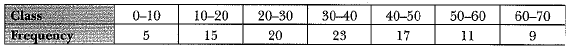Ans.
We have cumulative frequency table,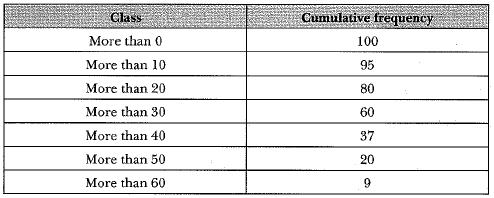N = 100
N/2=50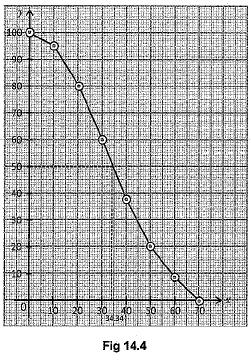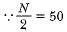∴ Median = 34.34 = 34.3

Q.5. The mean of the following distribution is 18. Find the frequency f of the class 19 - 21.    [CBSE 2018]
Ans.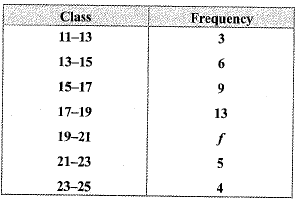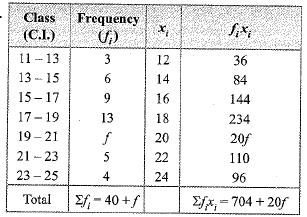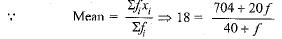⇒ 18(40+f) = 704+20f
⇒ 720+18f - 20f + 704
⇒ 720-704 = 20f - 18f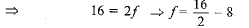Q.6. The following distribution gives the daily income of 50 workers of a factory:    [CBSE 2018]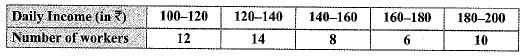Convert the distribution above to a less than type cumulative frequency distribution and draw its ogive.

Ans.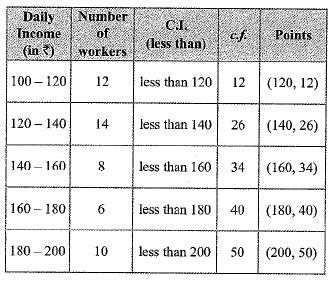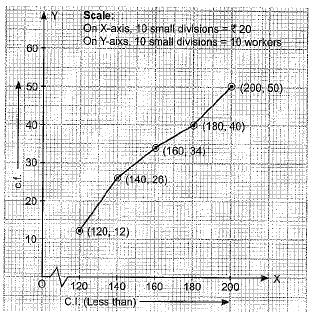Offer running on EduRev: Apply code STAYHOME200 to get INR 200 off on our premium plan EduRev Infinity!

## Mathematics (Maths) Class 10

62 videos|363 docs|103 tests

,

,

,

,

,

,

,

,

,

,

,

,

,

,

,

,

,

,

,

,

,

;